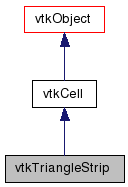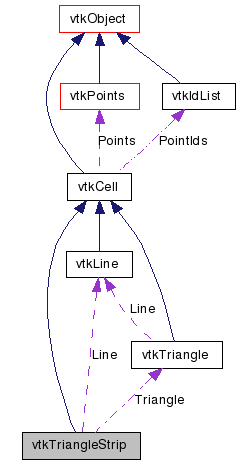# vtkTriangleStrip Class Reference

`#include <vtkTriangleStrip.h>`

Inheritance diagram for vtkTriangleStrip:[legend]
Collaboration diagram for vtkTriangleStrip:[legend]

## Detailed Description

a cell that represents a triangle strip

vtkTriangleStrip is a concrete implementation of vtkCell to represent a 2D triangle strip. A triangle strip is a compact representation of triangles connected edge to edge in strip fashion. The connectivity of a triangle strip is three points defining an initial triangle, then for each additional triangle, a single point that, combined with the previous two points, defines the next triangle.

Examples:
vtkTriangleStrip (Examples)
Tests:
vtkTriangleStrip (Tests)

Definition at line 41 of file vtkTriangleStrip.h.

## Public Types

typedef vtkCell Superclass

## Public Member Functions

virtual const char * GetClassName ()
virtual int IsA (const char *type)
void PrintSelf (ostream &os, vtkIndent indent)
int EvaluatePosition (double x, double *closestPoint, int &subId, double pcoords, double &dist2, double *weights)
void EvaluateLocation (int &subId, double pcoords, double x, double *weights)
int IntersectWithLine (double p1, double p2, double tol, double &t, double x, double pcoords, int &subId)
int Triangulate (int index, vtkIdList *ptIds, vtkPoints *pts)
void Derivatives (int subId, double pcoords, double *values, int dim, double *derivs)
int IsPrimaryCell ()
int GetParametricCenter (double pcoords)
int GetCellType ()
int GetCellDimension ()
int GetNumberOfEdges ()
int GetNumberOfFaces ()
vtkCellGetEdge (int edgeId)
vtkCellGetFace (int vtkNotUsed(faceId))
int CellBoundary (int subId, double pcoords, vtkIdList *pts)
void Contour (double value, vtkDataArray *cellScalars, vtkIncrementalPointLocator *locator, vtkCellArray *verts, vtkCellArray *lines, vtkCellArray *polys, vtkPointData *inPd, vtkPointData *outPd, vtkCellData *inCd, vtkIdType cellId, vtkCellData *outCd)
void Clip (double value, vtkDataArray *cellScalars, vtkIncrementalPointLocator *locator, vtkCellArray *polys, vtkPointData *inPd, vtkPointData *outPd, vtkCellData *inCd, vtkIdType cellId, vtkCellData *outCd, int insideOut)
virtual void InterpolateFunctions (double pcoords, double *weights)
virtual void InterpolateDerivs (double pcoords, double *derivs)

## Static Public Member Functions

static vtkTriangleStripNew ()
static int IsTypeOf (const char *type)
static vtkTriangleStripSafeDownCast (vtkObject *o)
static void DecomposeStrip (int npts, vtkIdType *pts, vtkCellArray *tris)

## Protected Member Functions

vtkTriangleStrip ()
~vtkTriangleStrip ()

## Protected Attributes

vtkLineLine
vtkTriangleTriangle

## Member Typedef Documentation

 typedef vtkCell vtkTriangleStrip::Superclass

Reimplemented from vtkCell.

Definition at line 45 of file vtkTriangleStrip.h.

## Constructor & Destructor Documentation

 vtkTriangleStrip::vtkTriangleStrip ( ) ` [protected]`

 vtkTriangleStrip::~vtkTriangleStrip ( ) ` [protected]`

## Member Function Documentation

 static vtkTriangleStrip* vtkTriangleStrip::New ( ) ` [static]`

Create an object with Debug turned off, modified time initialized to zero, and reference counting on.

Reimplemented from vtkObject.

 virtual const char* vtkTriangleStrip::GetClassName ( ) ` [virtual]`

Reimplemented from vtkCell.

 static int vtkTriangleStrip::IsTypeOf ( const char * name ) ` [static]`

Return 1 if this class type is the same type of (or a subclass of) the named class. Returns 0 otherwise. This method works in combination with vtkTypeMacro found in vtkSetGet.h.

Reimplemented from vtkCell.

 virtual int vtkTriangleStrip::IsA ( const char * name ) ` [virtual]`

Return 1 if this class is the same type of (or a subclass of) the named class. Returns 0 otherwise. This method works in combination with vtkTypeMacro found in vtkSetGet.h.

Reimplemented from vtkCell.

 static vtkTriangleStrip* vtkTriangleStrip::SafeDownCast ( vtkObject * o ) ` [static]`

Reimplemented from vtkCell.

 void vtkTriangleStrip::PrintSelf ( ostream & os, vtkIndent indent ) ` [virtual]`

Methods invoked by print to print information about the object including superclasses. Typically not called by the user (use Print() instead) but used in the hierarchical print process to combine the output of several classes.

Reimplemented from vtkCell.

 int vtkTriangleStrip::GetCellType ( ) ` [inline, virtual]`

See the vtkCell API for descriptions of these methods.

Implements vtkCell.

Definition at line 50 of file vtkTriangleStrip.h.

 int vtkTriangleStrip::GetCellDimension ( ) ` [inline, virtual]`

See the vtkCell API for descriptions of these methods.

Implements vtkCell.

Definition at line 51 of file vtkTriangleStrip.h.

 int vtkTriangleStrip::GetNumberOfEdges ( ) ` [inline, virtual]`

See the vtkCell API for descriptions of these methods.

Implements vtkCell.

Definition at line 52 of file vtkTriangleStrip.h.

 int vtkTriangleStrip::GetNumberOfFaces ( ) ` [inline, virtual]`

See the vtkCell API for descriptions of these methods.

Implements vtkCell.

Definition at line 53 of file vtkTriangleStrip.h.

 vtkCell* vtkTriangleStrip::GetEdge ( int edgeId ) ` [virtual]`

See the vtkCell API for descriptions of these methods.

Implements vtkCell.

 vtkCell* vtkTriangleStrip::GetFace ( int vtkNotUsedfaceId ) ` [inline]`

See the vtkCell API for descriptions of these methods.

Definition at line 55 of file vtkTriangleStrip.h.

 int vtkTriangleStrip::CellBoundary ( int subId, double pcoords, vtkIdList * pts ) ` [virtual]`

See the vtkCell API for descriptions of these methods.

Implements vtkCell.

 void vtkTriangleStrip::Contour ( double value, vtkDataArray * cellScalars, vtkIncrementalPointLocator * locator, vtkCellArray * verts, vtkCellArray * lines, vtkCellArray * polys, vtkPointData * inPd, vtkPointData * outPd, vtkCellData * inCd, vtkIdType cellId, vtkCellData * outCd ) ` [virtual]`

See the vtkCell API for descriptions of these methods.

Implements vtkCell.

 void vtkTriangleStrip::Clip ( double value, vtkDataArray * cellScalars, vtkIncrementalPointLocator * locator, vtkCellArray * polys, vtkPointData * inPd, vtkPointData * outPd, vtkCellData * inCd, vtkIdType cellId, vtkCellData * outCd, int insideOut ) ` [virtual]`

See the vtkCell API for descriptions of these methods.

Implements vtkCell.

 int vtkTriangleStrip::EvaluatePosition ( double x, double * closestPoint, int & subId, double pcoords, double & dist2, double * weights ) ` [virtual]`

Given a point x return inside(=1), outside(=0) cell, or (-1) computational problem encountered; evaluate parametric coordinates, sub-cell id (!=0 only if cell is composite), distance squared of point x to cell (in particular, the sub-cell indicated), closest point on cell to x (unless closestPoint is null, in which case, the closest point and dist2 are not found), and interpolation weights in cell. (The number of weights is equal to the number of points defining the cell). Note: on rare occasions a -1 is returned from the method. This means that numerical error has occurred and all data returned from this method should be ignored. Also, inside/outside is determine parametrically. That is, a point is inside if it satisfies parametric limits. This can cause problems for cells of topological dimension 2 or less, since a point in 3D can project onto the cell within parametric limits but be "far" from the cell. Thus the value dist2 may be checked to determine true in/out.

Implements vtkCell.

 void vtkTriangleStrip::EvaluateLocation ( int & subId, double pcoords, double x, double * weights ) ` [virtual]`

Determine global coordinate (x) from subId and parametric coordinates. Also returns interpolation weights. (The number of weights is equal to the number of points in the cell.)

Implements vtkCell.

 int vtkTriangleStrip::IntersectWithLine ( double p1, double p2, double tol, double & t, double x, double pcoords, int & subId ) ` [virtual]`

Intersect with a ray. Return parametric coordinates (both line and cell) and global intersection coordinates, given ray definition and tolerance. The method returns non-zero value if intersection occurs.

Implements vtkCell.

 int vtkTriangleStrip::Triangulate ( int index, vtkIdList * ptIds, vtkPoints * pts ) ` [virtual]`

Generate simplices of proper dimension. If cell is 3D, tetrahedron are generated; if 2D triangles; if 1D lines; if 0D points. The form of the output is a sequence of points, each n+1 points (where n is topological cell dimension) defining a simplex. The index is a parameter that controls which triangulation to use (if more than one is possible). If numerical degeneracy encountered, 0 is returned, otherwise 1 is returned. This method does not insert new points: all the points that define the simplices are the points that define the cell.

Implements vtkCell.

 void vtkTriangleStrip::Derivatives ( int subId, double pcoords, double * values, int dim, double * derivs ) ` [virtual]`

Compute derivatives given cell subId and parametric coordinates. The values array is a series of data value(s) at the cell points. There is a one-to-one correspondence between cell point and data value(s). Dim is the number of data values per cell point. Derivs are derivatives in the x-y-z coordinate directions for each data value. Thus, if computing derivatives for a scalar function in a hexahedron, dim=1, 8 values are supplied, and 3 deriv values are returned (i.e., derivatives in x-y-z directions). On the other hand, if computing derivatives of velocity (vx,vy,vz) dim=3, 24 values are supplied ((vx,vy,vz)1, (vx,vy,vz)2, ....()8), and 9 deriv values are returned ((d(vx)/dx),(d(vx)/dy),(d(vx)/dz), (d(vy)/dx),(d(vy)/dy), (d(vy)/dz), (d(vz)/dx),(d(vz)/dy),(d(vz)/dz)).

Implements vtkCell.

 int vtkTriangleStrip::IsPrimaryCell ( ) ` [inline, virtual]`

Return whether this cell type has a fixed topology or whether the topology varies depending on the data (e.g., vtkConvexPointSet). This compares to composite cells that are typically composed of primary cells (e.g., a triangle strip composite cell is made up of triangle primary cells).

Reimplemented from vtkCell.

Definition at line 79 of file vtkTriangleStrip.h.

 int vtkTriangleStrip::GetParametricCenter ( double pcoords ) ` [virtual]`

Return the center of the point cloud in parametric coordinates.

Reimplemented from vtkCell.

 static void vtkTriangleStrip::DecomposeStrip ( int npts, vtkIdType * pts, vtkCellArray * tris ) ` [static]`

Given a triangle strip, decompose it into a list of (triangle) polygons. The polygons are appended to the end of the list of triangles.

 virtual void vtkTriangleStrip::InterpolateFunctions ( double pcoords, double * weights ) ` [virtual]`

Compute the interpolation functions/derivatives (aka shape functions/derivatives)

 virtual void vtkTriangleStrip::InterpolateDerivs ( double pcoords, double * derivs ) ` [virtual]`

Compute the interpolation functions/derivatives (aka shape functions/derivatives)

## Member Data Documentation

 vtkLine* vtkTriangleStrip::Line` [protected]`

Definition at line 100 of file vtkTriangleStrip.h.

 vtkTriangle* vtkTriangleStrip::Triangle` [protected]`

Definition at line 101 of file vtkTriangleStrip.h.

The documentation for this class was generated from the following file:

Generated on Wed Aug 24 12:15:06 2011 for VTK by1.5.6